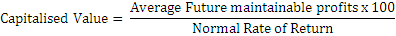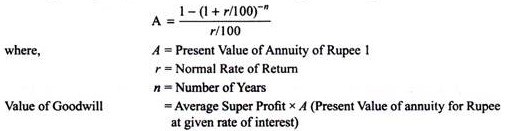# Explain various methods of valuation of goodwill

Explain various methods of valuation of goodwill

First of all, we have to understand about Goodwill. It is an intangible asset which represents non-physical of a company. It is of immense value but it is not easy to be identified or valued. Though it cannot be easily calculated, it’s no doubt that intangible assets significantly contribute to a company’s success and value.

There are several methods which can be implemented for valuation of goodwill which is as follows:

1. Average Profit Method

Goodwill’s value in this method is considered by multiplying the Average Future profit by a certain number of year’s purchase.

Goodwill = Future maintainable profit after tax x No. of years purchase

Steps Involved under Average Profits Method:

• Calculate past profits before tax.

• Calculate the future profit before tax after making past adjustments.

• Calculate the average past adjusted profits.

• Multiply the future profits to be maintained by the number of years’ purchase.

2. Super Profit Method:

This super profit method is the additional estimated future maintainable profits over the normal profits.

Steps Involved in Calculating Goodwill under Super Profit Method:

• Calculate capital employed (always should the aggregate of Shareholders’ equity and long-term debt or fixed assets and net current assets).

• Calculate the Usual Profits by multiplying employed capital with normal return rate.

• Calculate average maintainable profit.

• Calculate Super Profit as follows:

Super Profit = Maintainable Average profits – Normal Profits.

• Calculate goodwill by multiplying super profit by the number of year’s purchase.

3. Capitalization Method:

Goodwill under this method can be calculated by capitalizing average normal profit or capitalizing super profits. Following are those:-

(i) Capitalisation of Average Profit Method:

Under this particular system, goodwill can be discovered by deducting actual Capital Employed (i.e., valuation date of Net Assets) from the capitalised value of the average profits. It should be on the basis of the normal rate of Return (also known as the value of the firm or capitalised value of business)

Goodwill = Capitalised Value – Net Assets of Business

Following are the stages involved in calculating goodwill as per capitalisation of Average Profits Method:

• Calculate Average future maintainable profits

• Calculate the Capitalised value of business on the basis of the Average ProfitsCalculate the value of Net Assets on the valuation date

Net Assets means All Assets (other than fictitious assets, goodwill and non-trade investments) at their current values – Outsider’s Liabilities

• Calculate Goodwill (Goodwill equals to Capitalised Value – Net assets of the business.)

(ii) Capitalisation of Super Profit Method:

The goodwill assertion in this method is done by capitalizing the super profits on the basis of the normal rate of return. Capital needed for earning the super profit can be calculated by this method.

The value of goodwill is computed as follows:1. Annuity Method:

In the annuity method, goodwill can be calculated by taking average super profit. This particular profit is the value of an annuity over a certain number of years. Computation of the present value of this annuity is done by discounting it at the given rate of interest, i.e. on the normal rate of return. Valuation of goodwill is this discounted present value of the annuity.

If the value of the annuity is not given, it can be calculated with the help of following formula: